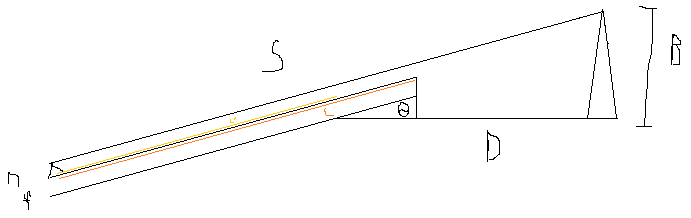# Guitar Neck Angle

The neck angle on a guitar is an issue that has confused me.  I think the issue is that most guitar building books are aimed at a general audience who is not assumed to have any knowledge of math, while I sometimes find that a little math can clarify things.

So, for example, when a book mentions figuring out the neck angle, they mention making a drawing to scale to be sure you have it right.  Okay, but suppose I’d like to know general trends.  Changing the bridge height by some amount changes the angle by…?So the above picture is the set up of the problem.  We have the bridge height (B), fretboard height (f), string height at nut (n), and distance from where the fretboard joins the body to the bridge (D).

Going through the geometry, we getThis is a somewhat complicated equation for theta, but if we take the first order taylor expansion, we get a simple solution:So, generally, a higher bridge, all else being equal, will need a larger neck angle.

Let’s do an example.  One book says that a typical bridge height is from 3/8 to 1/2″, but let’s go to 3/4″.  Fretboard height will be about 1/4″.  Nut height might be about 0.07″.

From the image in an earlier post, the neck joins the body at the 19th fret.  This means d = 5.005″.

(In fact, since the scale length is parallel to the strings, not the body, d itself could be corrected here, based on the angle.  We’ll ignore that for now)

Based on the extremes in bridge heights, the angle could be from 0.010989 to 0.08591. About 0.6 to 4.9 degrees.

And if we solved that earlier equation numerically?  0.010984 to 0.08547.  At most, the simpler equation gives an error of about 0.5%, or 0.02 degrees.  A scale, by-hand drawing certainly won’t be that accurate.

As far as correcting d goes,to first order, instead of d = S – L’, it’s d = S – (L’ + n theta).  So d might go from 5.005 to 4.9997.  Of course, the adjustability in the bridge should take care of that.

(And you could treat the equations for d and b as coupled equations in d and theta, but the correction that adds will also be very small)

Another thing I was thinking about was, What should the clearance of the string be at the first fret past where it’s fretted?  As you move up the neck, the angle becomes steeper, but on the other hand the distance to the next fret decreases.  It turns out these two effects cancel, and the string clearance is constant.  It’s 1 – 1/2^(1/12), or about 0.05 times the clearance of the unfretted string.

## One thought on “Guitar Neck Angle”

1.admin on said:

Of course, that’s only true if the action is constant (as in the drawings). If the action towards the bridge end is higher than towards the nut, then the clearance when fretted near the nut will be greater than when fretted higher up the neck.